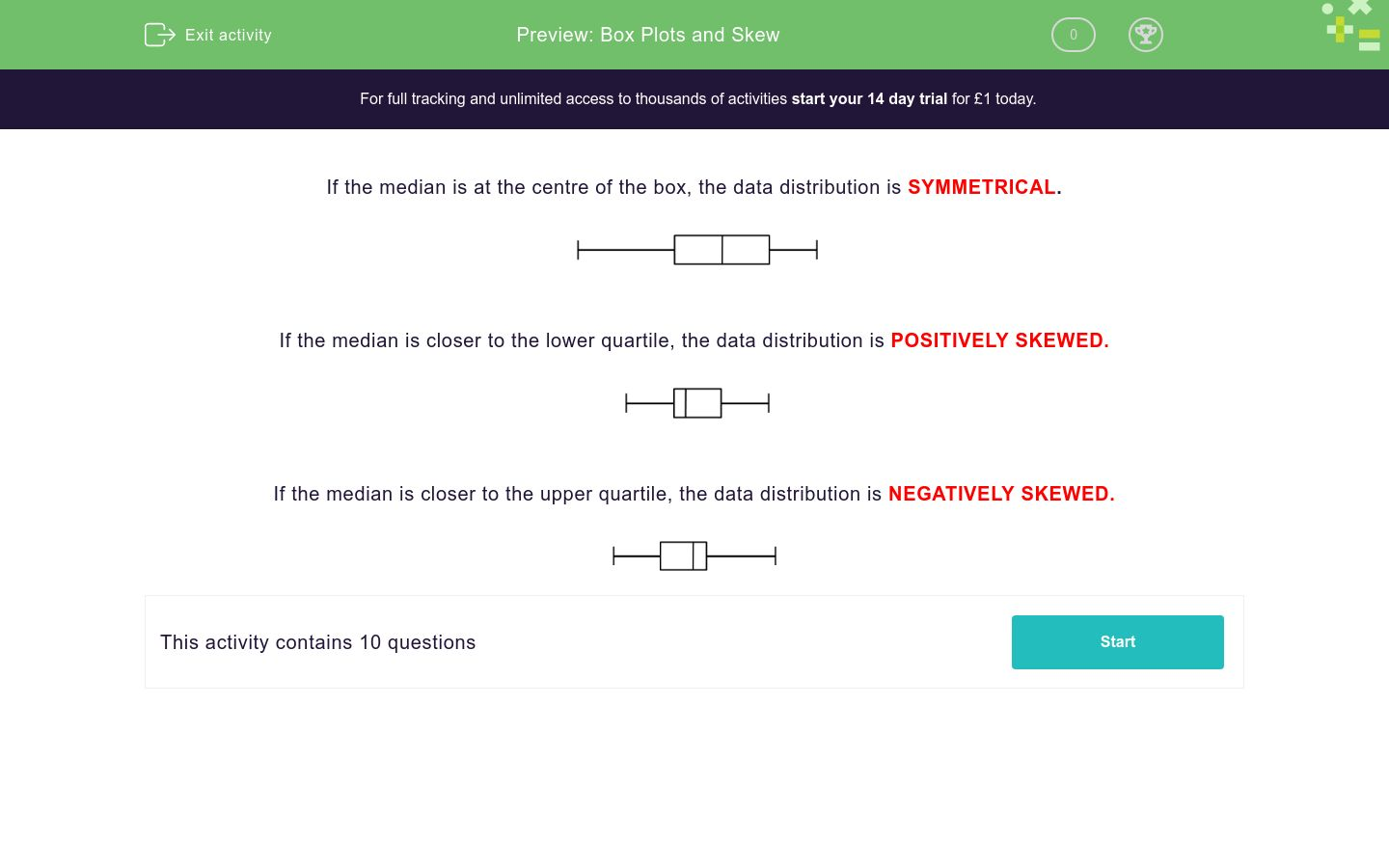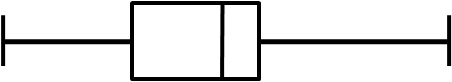# Box Plots and Skew

In this worksheet, students identify symmetrical, positively skewed and negatively skewed data from box plot diagrams.Key stage:  KS 4

Curriculum topic:  Statistics

Difficulty level:### QUESTION 1 of 10

If the median is at the centre of the box, the data distribution is SYMMETRICAL.If the median is closer to the lower quartile, the data distribution is POSITIVELY SKEWED.If the median is closer to the upper quartile, the data distribution is NEGATIVELY SKEWED.From the information in this box plot diagram, state how the distribution is skewed or if it is symmetrical.symmetrical

positively skewed

negatively skewed

From the information in this box plot diagram, state how the distribution is skewed or if it is symmetrical.symmetrical

positively skewed

negatively skewed

From the information in this box plot diagram, state how the distribution is skewed or if it is symmetrical.symmetrical

positively skewed

negatively skewed

From the information in this box plot diagram, state how the distribution is skewed or if it is symmetrical.symmetrical

positively skewed

negatively skewed

From the information in this box plot diagram, state how the distribution is skewed or if it is symmetrical.symmetrical

positively skewed

negatively skewed

From the information in this box plot diagram, state how the distribution is skewed or if it is symmetrical.symmetrical

positively skewed

negatively skewed

From the information in this box plot diagram, state how the distribution is skewed or if it is symmetrical.symmetrical

positively skewed

negatively skewed

From the information in the box plot diagram labelled A, state how the distribution is skewed or if it is symmetrical.symmetrical

positively skewed

negatively skewed

From the information in the box plot diagram labelled B, state how the distribution is skewed or if it is symmetrical.symmetrical

positively skewed

negatively skewed

From the information in the box plot diagram labelled C, state how the distribution is skewed or if it is symmetrical.symmetrical

positively skewed

negatively skewed

• Question 1

From the information in this box plot diagram, state how the distribution is skewed or if it is symmetrical.positively skewed
EDDIE SAYS
The median is closer to the lower quartile.
• Question 2

From the information in this box plot diagram, state how the distribution is skewed or if it is symmetrical.negatively skewed
EDDIE SAYS
The median is closer to the upper quartile.
• Question 3

From the information in this box plot diagram, state how the distribution is skewed or if it is symmetrical.symmetrical
EDDIE SAYS
The median is halfway between the lower and upper quartiles.
• Question 4

From the information in this box plot diagram, state how the distribution is skewed or if it is symmetrical.positively skewed
EDDIE SAYS
The median is closer to the lower quartile.
• Question 5

From the information in this box plot diagram, state how the distribution is skewed or if it is symmetrical.negatively skewed
EDDIE SAYS
The median is closer to the upper quartile.
• Question 6

From the information in this box plot diagram, state how the distribution is skewed or if it is symmetrical.negatively skewed
EDDIE SAYS
The median is closer to the upper quartile.
• Question 7

From the information in this box plot diagram, state how the distribution is skewed or if it is symmetrical.negatively skewed
EDDIE SAYS
The median is closer to the upper quartile.
• Question 8

From the information in the box plot diagram labelled A, state how the distribution is skewed or if it is symmetrical.positively skewed
EDDIE SAYS
The median is closer to the lower quartile.
• Question 9

From the information in the box plot diagram labelled B, state how the distribution is skewed or if it is symmetrical.symmetrical
EDDIE SAYS
The median is in the centre of the box.
• Question 10

From the information in the box plot diagram labelled C, state how the distribution is skewed or if it is symmetrical.negatively skewed
EDDIE SAYS
The median is closer to the upper quartile.
---- OR ----

Sign up for a £1 trial so you can track and measure your child's progress on this activity.

### What is EdPlace?

We're your National Curriculum aligned online education content provider helping each child succeed in English, maths and science from year 1 to GCSE. With an EdPlace account you’ll be able to track and measure progress, helping each child achieve their best. We build confidence and attainment by personalising each child’s learning at a level that suits them.